﻿ 解决pandas的concat函数导致索引失效的方法
﻿

# concat

``````for i, bag in enumerate(bags):

coure_result = func()
core_df = pd.DataFrame([core_result])

dfs.append(core_df)
df = pd.concat(dfs)
``````

``````df_summary = pd.DataFrame(summary, columns = ["summary"])
df.insert(1,"summary",df_summary["summary"])
``````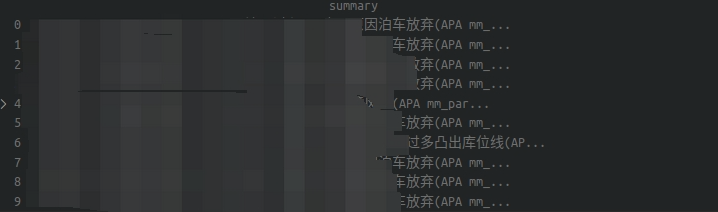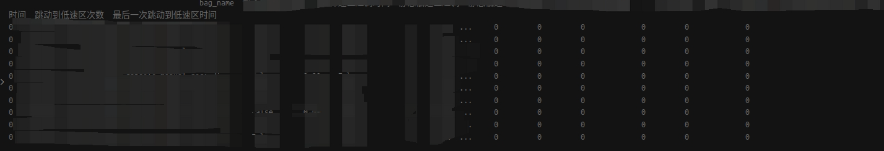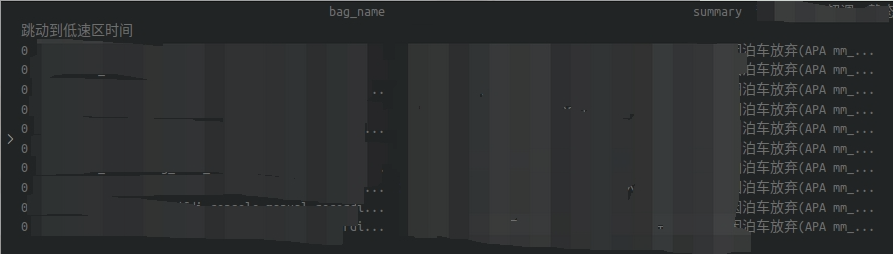``````df = pd.concat(dfs).reset_index(drop = True)
``````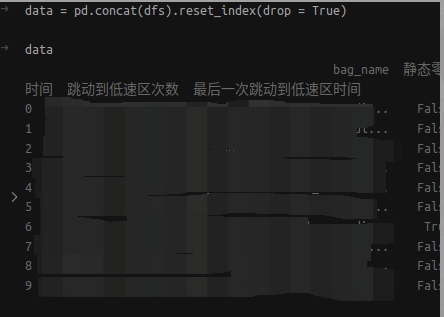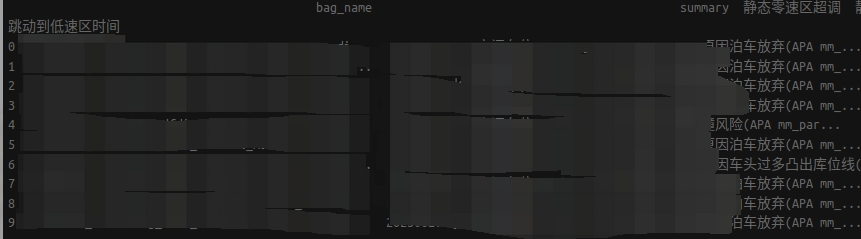__EOF__

• 本文作者： IvanLee
• 本文链接： https://www.cnblogs.com/ivanlee717/p/17514757.html

﻿
相关教程